# Discretely-normed ring

(Redirected from Discrete valuation ring)

discrete valuation ring, discrete valuation domain

A ring with a discrete valuation, i.e. an integral domain with a unit element in which there exists an elementsuch that any non-zero ideal is generated by some power of the element; such an element is called a uniformizing parameter, and is defined up to multiplication by an invertible element. Each non-zero element of a discretely-normed ring can be uniquely written in the form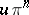, whereis an invertible element andis an integer. Examples of discretely-normed rings include the ring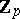of-adic integers, the ringof formal power series in one variableover a field, and the ring of Witt vectors (cf. Witt vector)for a perfect field.

A discretely-normed ring may also be defined as a local principal ideal ring; as a local one-dimensional Krull ring; as a local Noetherian ring with a principal maximal ideal; as a Noetherian valuation ring; or as a valuation ring with group of values.

The completion (in the topology of a local ring) of a discretely-normed ring is also a discretely-normed ring. A discretely-normed ring is compact if and only if it is complete and its residue field is finite; any such ring is either isomorphic to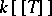, whereis a finite field, or else is a finite extension of.

Ifis a local homomorphism of discretely-normed rings with uniformizing elementsand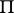, then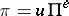, whereis an invertible element in. The integer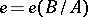is the ramification index of the extension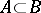, and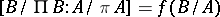is called the residue degree. This situation arises when one considers the integral closureof a discretely-normed ringwith a field of fractionsin a finite extensionof. In such a caseis a semi-local principal ideal ring; if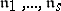are its maximal ideals, then the localizations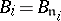are discretely-normed rings. Ifis a separable extension ofof degree, the formula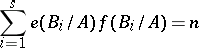is valid. If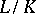is a Galois extension, then alland all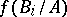are equal, and. Ifis a complete discretely-normed ring,itself will be a discretely-normed ring and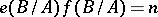. On these assumptions the extension(and alsoover) is known as an unramified extension ifand the field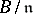is separable over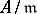; it is weakly ramified if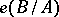is relatively prime with the characteristic of the fieldwhileis separable over; it is totally ramified if.

The theory of modules over a discretely-normed ring is very similar to the theory of Abelian groups . Any module of finite type is a direct sum of cyclic modules; a torsion-free module is a flat module; any projective module or submodule of a free module is free. However, the direct product of an infinite number of free modules is not free. A torsion-free module of countable rank over a complete discretely-normed ring is a direct sum of modules of rank one.